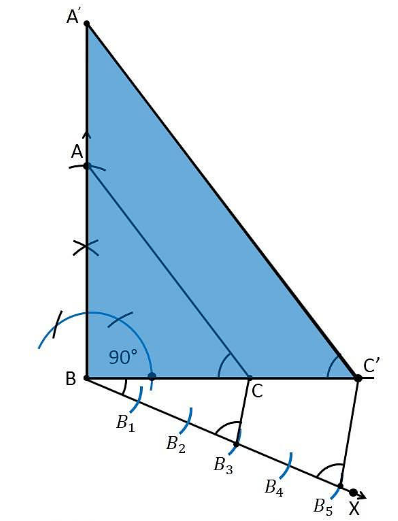Guru

# Draw a right triangle in which the sides (other than hypotenuse) are of lengths 4 cm and 3 cm. Then construct another triangle whose sides are 5/3 times the corresponding sides of the given triangle. Q.7

• 0

Sir give me the best and simple way to solve the problem of question from class 10th ncert of constructions chapter of exercise 11.1 of question no.7, how i solve this problem Draw a right triangle in which the sides (other than hypotenuse) are of lengths 4 cm and 3 cm. Then construct another triangle whose sides are 5/3 times the corresponding sides of the given triangle.

Share

1. The sides other than hypotenuse are of lengths 4cm and 3cm. It defines that the sides are perpendicular to each other

Construction Procedure:

The required triangle can be drawn as follows.

1. Draw a line segment BC =3 cm.

2. Now measure and draw ∠= 90°

3. Take B as centre and draw an arc with the radius of 4 cm and intersects the ray at the point B.

4. Now, join the lines AC and the triangle ABC is the required triangle.

5. Draw a ray BX makes an acute angle with BC on the opposite side of vertex A.

6. Locate 5 such as B1, B2, B3, B4, on the ray BX such that such that BB1 = B1B2 = B2B3= B3B4 = B4B5

7. Join the points B3C.

8. Draw a line through B5 parallel to B3C which intersects the extended line BC at C’.

9. Through C’, draw a line parallel to the line AC that intersects the extended line AB at A’.

10. Therefore, ΔA’BC’ is the required triangle.Justification:

The construction of the given problem can be justified by proving that

Since the scale factor is 5/3, we need to prove

A’B = (5/3)AB

BC’ = (5/3)BC

A’C’= (5/3)AC

From the construction, we get A’C’ || AC

In ΔA’BC’ and ΔABC,

∴ ∠ A’C’B = ∠ACB (Corresponding angles)

∠B = ∠B (common)

∴ ΔA’BC’ ∼ ΔABC (From AA similarity criterion)

Since the corresponding sides of the similar triangle are in the same ratio, it becomes

Therefore, A’B/AB = BC’/BC= A’C’/AC

So, it becomes A’B/AB = BC’/BC= A’C’/AC = 5/3

Hence, justified.

• 0